Thank you for visiting nature.com. You are using a browser version with limited support for CSS. To obtain the best experience, we recommend you use a more up to date browser (or turn off compatibility mode in Internet Explorer). In the meantime, to ensure continued support, we are displaying the site without styles and JavaScript.

# Dynamical synapses causing self-organized criticality in neural networks

## Abstract

Self-organized criticality1 is one of the key concepts to describe the emergence of complexity in natural systems. The concept asserts that a system self-organizes into a critical state where system observables are distributed according to a power law. Prominent examples of self-organized critical dynamics include piling of granular media2, plate tectonics3 and stick–slip motion4. Critical behaviour has been shown to bring about optimal computational capabilities5, optimal transmission6, storage of information7 and sensitivity to sensory stimuli8,9,10. In neuronal systems, the existence of critical avalanches was predicted11 and later observed experimentally6,12,13. However, whereas in the experiments generic critical avalanches were found, in the model of ref. 11 they only show up if the set of parameters is fine-tuned externally to a critical transition state. Here, we demonstrate analytically and numerically that by assuming (biologically more realistic) dynamical synapses14 in a spiking neural network, the neuronal avalanches turn from an exceptional phenomenon into a typical and robust self-organized critical behaviour, if the total resources of neurotransmitter are sufficiently large.

## Main

In multi-electrode recordings on slices of rat cortex and neuronal cultures6,12, neuronal avalanches were observed whose sizes were distributed according to a power law with an exponent of −3/2. The distribution was stable over a long period of time. Variations of the dynamical behaviour are induced by application or wash-out of neuromodulators. Qualitatively identical behaviour can be reached in models such as those in refs 11,15 by variations of a global connectivity parameter. In these models, criticality only shows up if the interactions are fixed precisely at a specific value or connectivity structure.

Here, we study a model with activity-dependent depressive synapses and show that existence of several dynamical regimes can be reconciled with parameter-independent criticality. We find that synaptic depression causes the mean synaptic strengths to approach a critical value for a certain range of interaction parameters, whereas outside this range other dynamical behaviours are prevalent, see Fig. 1. We analytically derive an expression for the average coupling strengths among neurons and the average inter-spike intervals in a mean-field approach. The mean-field approximation is applicable here even in the critical state, because the quantities that are averaged do not exhibit power laws, but unimodal distributions. These mean values obey a self-consistency equation that allows us to identify the mechanism that drives the dynamics of the system towards the critical regime. Moreover, the critical regime induced by the synaptic dynamics is robust to parameter changes.Figure 1: Distribution of avalanche sizes for different coupling strengths α.

Consider a network of N integrate-and-fire neurons. Each neuron is characterized by a membrane potential 0<hi(t)<θ. The neurons receive external inputs by a random process ξτ{1,…,N} that selects a neuron ξτ(t)=i at a rate τ and advances the membrane potential hi by an amount Iext. Each neuron integrates inputs until it reaches a threshold θ. As soon as hi(t)>θ, the neuron emits a spike that is delivered to all postsynaptic neurons at a fixed delay τd. The membrane potential is reset by hi(tsp+)=hi(tsp)−θ. For simplicity we will assume in the following that θ=1. Super-threshold activity is communicated along neural connections of a strength proportional to Ji j to other neurons and may cause them to fire. In this way an avalanche of neural activity of size L≥1 is triggered. More precisely, an avalanche is a period of activity that is initiated by an external input and that terminates when no further neuron becomes activated. We define the size of the avalanche as the number of participating neurons. The dynamics of the membrane potential is described by the following equation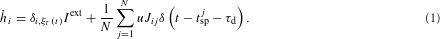In studies of self-organized criticality typically a separation of timescales is assumed which enters in equation (1) by the condition τdτ. It allows us to assume that external input is absent during avalanches. Later, in the discrete version of the model, τ will play the role of the temporal step size. The variables Ji j are subject to the dynamics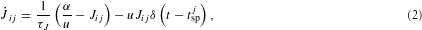which describes the amount of available neurotransmitter in the corresponding synapse14. Namely, if a spike arrives at the synapse, the available transmitter is diminished by a fraction u, that is, the synaptic strength decreases owing to the use of transmitter resources. If the presynaptic neuron is silent then the synapse recovers and its strength Ji j approaches the value α/u at a slow timescale τJ=τ ν N with 1<νN. Thus, the maximal strength of a connection is determined by the parameter α and can be observed only when the synapse is fully recovered. The behaviour of the network is determined, however, by the averaged synaptic strength which will be denoted by 〈Ji j〉 with the average taken with respect to the distribution of inter-spike intervals. To obtain our main result we will calculate this effective value and use it in a static network. The uniform strengths of the static network are denoted by α0.

If the external drive and the synaptic weights are small, the activity of the network consists of short burst-like events that are initiated by a particular external input. The firing events are separated by relatively long relaxation intervals when external inputs are integrated. We may thus be tempted to assume Jα/u before any spiking event. In general, however, we must take into account that the efficacy of a synapse varies in a use-dependent way which compensates large levels of network activity. Depending on the synaptic strength, the network can produce a rich repertoire of behaviours. In Fig. 1, we show examples of avalanche-size distributions for various values of α. For small values of α, subcritical avalanche-size distributions are observed. This regime is characterized by a negligible number of avalanches that extend to the system size. For larger values of α, the system develops an approximate power-law distribution for avalanche sizes almost up to the system size where an exponential cutoff is observed. The mean-squared deviation from an exact power law is shown in Fig. 2. Finally, the distribution of avalanche sizes becomes non-monotonous when α is well above the critical region.Figure 2: The range of connectivity parameters that cause a critical dynamics extends with system size.

In preliminary numerical studies we had assumed a model with facilitating and depressing synapses 16. Here, we conclude that facilitating synapses are not necessary to evoke self-organized critical avalanches in spiking neural networks, depressing synapses are sufficient. This is in line with the observation17 that synapses that connect excitatory neurons are largely depressive. To identify the parameters of the avalanche-size distribution it is sufficient to determine the average synaptic strength: as seen in Fig. 3 both the power-law exponent and the mean-squared deviation from the power law are the same for networks with dynamical synapses and networks with static synapses if the strength of the static synapses is chosen as α0=uJi j〉. To calculate the average synaptic strength analytically, we consider in addition the neural inter-spike intervals Δisi. On the one hand, if the inter-spike intervals are short then the synapses have a short time to recover and the average synaptic strength resides at a low level. On the other hand, large synaptic strengths lead to long avalanches and to large input to neurons during the avalanches, which tends to shorten the inter-spike intervals.

This trade-off determines the expected values of the synaptic strengths and the inter-spike intervals which are realized by the dynamics of the network. To express this reasoning more formally, we solve the dynamical equations (1) and (2) on the basis of a stationarity assumption for both the synaptic strengths and the inter-spike interval. Neither of these quantities has a power-law distribution and their first moments exist. In the Methods section, we derive expressions of the mean synaptic strength 〈Ji j〉 and the mean value of the inter-spike intervals distribution 〈Δisi〉. The stochastic dependency of the two quantities is reflected in a mutual dependence of their averages. Each of the dependencies is derived analytically, which allows us to formulate the self-consistency of the stationarity requirement as the simultaneous solution of the mean-field equations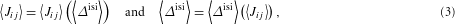which can be determined graphically from the intersections of the solutions of equations (7) and (12), see Fig. 4. The mean-field solution is confirmed by direct network simulations that are represented by the circles in Fig. 4. The solution is unique for any α. The stationary distribution is less sensitive to changes of the parameter α near the critical value of the synaptic strength than further away from it. This brings about the large critical region for the model with depressive synapses, see Fig. 2.Figure 4: The graphical solution of equation (3) establishes a functional relation between the average synaptic strength and inter-spike interval.

Furthermore, we want to discuss the stability of the solution of the self-consistency equation (3). If we apply a perturbation ΔJ to all synapses at time tp such that for each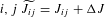, we can show by some simple computations that before the next spike the synaptic strengths are on average smaller than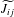. In the simulated system, the average synaptic strength is driven back to the fixed point by a few spikes, such that the solution of equation (3) is indeed stable for the critical state.

Both the numerical study in ref. 16 and the analysis presented so far refer to finite systems. The inset of Fig. 2 suggests that the critical region grows with increasing network size. This observation can be verified by considering the behaviour of the mean synaptic strength in the thermodynamic limit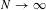. We compute the expectation value of the avalanche-size distribution (11), 〈L〉=N/(N−(N−1)α0) (ref. 11), and insert it into the self-consistency equation (3)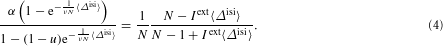In the limit, we should scale the external input by IextNw with w>0. We now distinguish the following cases. (1) If 〈ΔisiN1+ε and ε>w, then the right-hand side of equation (4) tends to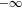, whereas the left-hand side is always larger than 0. (2) If 〈ΔisiN1+ε and 0<εw, then the right-hand side of equation (4) tends to 1 (or to 0 for ε=w), whereas the left-hand side approaches α; hence, a solution is only possible if α=1 and in this case uJi j〉→1. (3) If 〈ΔisiN1+ε and ε<0, then the right-hand side of equation (4) tends to 1, whereas the left-hand side approaches 0. (4) If 〈ΔisiN, we can assume that 〈Δisi〉=c N+o(N). From equation (4), we find the unique solution c=−ν(ln(α−1)−ln(α−1+u)) for α>1 and τJ=ν N, if we choose a time step of width τ. In all cases where a solution exists, we find uJi j〉→1, which is the critical connectivity for the network with static synapses in the limit. Therefore, in the thermodynamic limit the network with dynamical synapses becomes critical for any α≥1.

Here, we have focused on fully connected networks and neurons without leak currents for reasons of analytical tractability. We now discuss the results of various generalizations that we have investigated numerically. If the network has only partial connectivity, the results stay the same if the synaptic strengths are properly rescaled. In a random network of size N with connectivity probability c, the critical parameter α is approximately equal to αNcr/c, where αNcr is obtained from the critical parameter region of the fully connected network of size c×N. If the connections in a partially connected random network are not chosen independently (for example, ‘small-world’ connectivity18), even more accurate power laws than for the independent case with the same average connectivity are found. A similar phenomenon occurs in the grid network19 which has been used to model criticality in electroencephalograph recordings.

If in equation (1) we add a leak term, which is present in biologically realistic situations,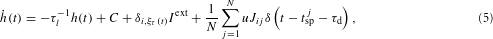we find numerically that the distribution of the avalanche sizes remains a power law for leak time constants up to τl≈40 ms. In equation (5) we included a constant compensatory synaptic current C that depends on τl and summarizes neuronal self-regulatory mechanisms. In this way, the probability of the neuron to stay near threshold is conserved and avalanches are triggered in a similar way as in the non-leaky case. The resulting power-law exponent is slightly smaller than −1.5 and reaches values close to −2 for strong leakage in simulations of a network of N=500 neurons.

In summary, we have presented an analytical and numerical study of spiking neural networks with dynamical synapses. Activity-dependent synaptic regulation leads to the self-organization of the network towards a critical state. The analysis demonstrates that mean synaptic efficacy hereby plays a crucial role. We explained how the critical state depends on the maximally available resources and have shown that in the thermodynamic limit the network becomes critical for any α≥1, that is, that criticality is an intrinsic phenomenon produced by the synaptic dynamics. The mean-field quantities are in very good agreement with simulations and were shown to be robust with respect to perturbations of the model parameters.

## Methods

In this section we give an explicit derivation of the self-consistency relation equation (3). Solving equation (2) between two spikes of neuron j we find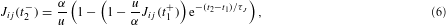where the synaptic strengths immediately before and after a spike of neuron j at time ts are denoted by Ji j(ts) and Ji j(ts+), respectively. Within a short interval containing the spike, Ji j decreases by a fraction u such that Ji j(t1+)=(1−u)Ji j(t1).

The average synaptic strength 〈Ji j〉 is the expectation value of Ji j(ts). Analogously, 〈Δisi〉 refers to the inter-spike interval Δisi. The random variables Ji j(ts) and Δisi both depend on the distribution of external inputs and are thus related. The self-consistency conditions equation (3) express this relation for the respective averages. Assuming that 〈Ji j〉 depends essentially only on the mean inter-spike interval, we obtain from equation (6):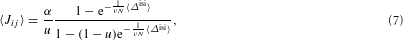where the average is carried out in discrete time with step width τ, that is, τJ=ν N.

We are now going to establish a relation between P(Δisi) and the inter-avalanche interval distribution Q(Δiai). It can be shown that the neuronal membrane potentials before an avalanche are uniformly distributed on the interval [εN,θ], where εN is a lower bound of hi(tsp)−θ with εN→0 for. Under these conditions, Q(Δiai) has a geometric distribution,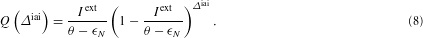Let kj be the number of avalanches between two spikes of the neuron j. A mean-field approximation relates the averages of the distributions of inter-spike and inter-avalanche intervals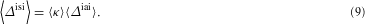The average inter-avalanche interval is easily computed from equation (8)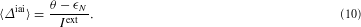To calculate the average number of avalanches between two spikes of a neuron, we compute the time to reach the threshold by accumulating external inputs and spikes from other neurons during avalanches, that is,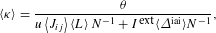where 〈L〉 is the mean avalanche size and 1/N is the probability that neuron j is receiving an input. The distribution of avalanche sizes can be computed analytically for a network with static synapses of strength α0 (ref. 11):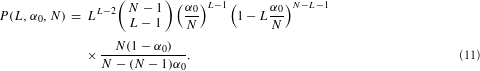In the case of dynamical synapses, we apply a mean-field approximation and set α0=uJi j〉 in equation (11). This allows us to compute 〈L〉 as a function of (uJi j〉).

Combining equations (9)–(11), we obtain a relation between the inter-spike interval and the average synaptic strength.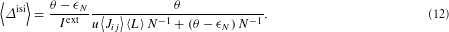The self-consistency equation (3) arises from equations (7) and (12). Its solution is obtained by numerical analysis of the two independent relations equations (7) and (12), see Fig. 4.

## References

1. 1

Bak, P., Tang, C. & Wiesenfeld, K. Self-organized criticality: An explanation of the 1/f noise. Phys. Rev. Lett. 59, 381–384 (1987).

2. 2

Frette, V. et al. Avalanche dynamics in a pile of rice. Nature 397, 49–52 (1996).

3. 3

Gutenberg, B. & Richter, C. F. Magnitude and energy of earthquakes. Ann. Geofis. 9, 1–15 (1956).

4. 4

Feder, H. J. S. & Feder, J. Self-organized criticality in a stick-slip process. Phys. Rev. Lett. 66, 2669–2672 (1991).

5. 5

Legenstein, R. & Maass, W. Edge of chaos and prediction of computational performance for neural circuit models. Neural Networks 20, 323–334 (2007).

6. 6

Beggs, J. & Plenz, D. Neuronal avalanches in neocortical circuits. J. Neurosci. 23, 11167–11177 (2003).

7. 7

Haldeman, C. & Beggs, J. Critical branching captures activity in living neural networks and maximizes the number of metastable states. Phys. Rev. Lett. 94, 058101 (2005).

8. 8

Der, R., Hesse, F. & Martius, G. Rocking stamper and jumping snake from a dynamical system approach to artificial life. Adapt. Behav. 14, 105–115 (2006).

9. 9

Kinouchi, O. & Copelli, M. Optimal dynamical range of excitable networks at criticality. Nature Phys. 2, 348–352 (2006).

10. 10

Chialvo, D. Are our senses critical? Nature Phys. 2, 301–302 (2006).

11. 11

Eurich, C. W., Herrmann, M. & Ernst, U. Finite-size effects of avalanche dynamics. Phys. Rev. E 66, 066137 (2002).

12. 12

Beggs, J. & Plenz, D. Neuronal avalanches are diverse and precise activity patterns that are stable for many hours in cortical slice cultures. J. Neurosci. 24, 5216–5229 (2004).

13. 13

Plenz, D. & Thiagarajan, T. C. The organizing principles of neuronal avalanches: cell assemblies in the cortex? Trends Neurosci. 30, 101–110 (2007).

14. 14

Markram, H. & Tsodyks, M. Redistribution of synaptic efficacy between pyramidal neurons. Nature 382, 807–810 (1996).

15. 15

Teramae, J. & Fukai, T. Local cortical circuit model inferred from power-law distributed neuronal avalanches. J. Comput. Neurosci. 22, 301–312 (2007).

16. 16

Levina, A., Herrmann, J. M. & Geisel, T. in Advances in Neural Information Processing Systems 18 (eds Weiss, Y., Schölkopf, B. & Platt, J.) 771–778 (MIT Press, Cambridge, 2006).

17. 17

Tsodyks, M., Pawelzik, K. & Markram, H. Neural networks with dynamic synapses. Neural Comput. 10, 821–835 (1998).

18. 18

Lin, M. & Chen, T.-L. Self-organized criticality in a simple model of neurons based on small-world networks. Phys. Rev. E 71, 016133 (2005).

19. 19

de Arcangelis, L., Perrone-Capano, C. & Herrmann, H. J. Self-organized criticality model for brain plasticity. Phys. Rev. Lett. 96, 028107 (2006).

## Acknowledgements

We thank M. Denker and T. Fukai for useful discussions. This work was partially supported by the BMBF in the framework of the Bernstein Centers for Computational Neuroscience, grant number 01GQ0432. A.L. has received support from DFG Graduiertenkolleg N1023.

## Author information

Authors

### Corresponding author

Correspondence to J. M. Herrmann.

## Rights and permissions

Reprints and Permissions

Levina, A., Herrmann, J. & Geisel, T. Dynamical synapses causing self-organized criticality in neural networks. Nature Phys 3, 857–860 (2007). https://doi.org/10.1038/nphys758

• Accepted:

• Published:

• Issue Date:

• ### A novel methodology to describe neuronal networks activity reveals spatiotemporal recruitment dynamics of synchronous bursting states

• Mallory Dazza
• , Stephane Métens
• , Pascal Monceau
•  & Samuel Bottani

Journal of Computational Neuroscience (2021)

• ### Mesoscopic population equations for spiking neural networks with synaptic short-term plasticity

• Valentin Schmutz
• , Wulfram Gerstner
•  & Tilo Schwalger

The Journal of Mathematical Neuroscience (2020)

• ### Fingerprints of a second order critical line in developing neural networks

• Karlis Kanders
• , Hyungsub Lee
• , Nari Hong
• , Yoonkey Nam
•  & Ruedi Stoop

Communications Physics (2020)

• ### Control of criticality and computation in spiking neuromorphic networks with plasticity

• Benjamin Cramer
• , David Stöckel
• , Markus Kreft
• , Michael Wibral
• , Johannes Schemmel
• , Karlheinz Meier
•  & Viola Priesemann

Nature Communications (2020)

• ### Network science characteristics of brain-derived neuronal cultures deciphered from quantitative phase imaging data

• Chenzhong Yin
• , Xiongye Xiao
• , Valeriu Balaban
• , Mikhail E. Kandel
• , Young Jae Lee
• , Gabriel Popescu
•  & Paul Bogdan

Scientific Reports (2020)

## Search